## Modelica.Blocks.Sources

Library of signal source blocks generating Real and Boolean signals

### Information

This package contains source components, i.e., blocks which have only output signals. These blocks are used as signal generators for Real, Integer and Boolean signals.

All Real source signals (with the exception of the Constant source) have at least the following two parameters:

 offset Value which is added to the signal startTime Start time of signal. For time < startTime, the output y is set to offset.

The offset parameter is especially useful in order to shift the corresponding source, such that at initial time the system is stationary. To determine the corresponding value of offset, usually requires a trimming calculation.

Extends from Modelica.Icons.SourcesPackage (Icon for packages containing sources).

### Package Content

NameDescriptionRealExpression Set output signal to a time varying Real expression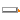IntegerExpression Set output signal to a time varying Integer expression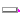BooleanExpression Set output signal to a time varying Boolean expression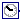Clock Generate actual time signalConstant Generate constant signal of type RealStep Generate step signal of type RealRamp Generate ramp signalSine Generate sine signalCosine Generate cosine signal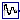ExpSine Generate exponentially damped sine signalExponentials Generate a rising and falling exponential signalPulse Generate pulse signal of type Real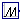SawTooth Generate saw tooth signalTrapezoid Generate trapezoidal signal of type RealKinematicPTP Move as fast as possible along a distance within given kinematic constraintsKinematicPTP2 Move as fast as possible from start to end position within given kinematic constraints with output signals q, qd=der(q), qdd=der(qd)TimeTable Generate a (possibly discontinuous) signal by linear interpolation in a tableCombiTimeTable Table look-up with respect to time and linear/periodic extrapolation methods (data from matrix/file)BooleanConstant Generate constant signal of type Boolean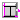BooleanStep Generate step signal of type Boolean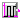BooleanPulse Generate pulse signal of type BooleanSampleTrigger Generate sample trigger signalBooleanTable Generate a Boolean output signal based on a vector of time instantsRadioButtonSource Boolean signal source that mimics a radio buttonIntegerConstant Generate constant signal of type IntegerIntegerStep Generate step signal of type IntegerIntegerTable Generate an Integer output signal based on a table matrix with [time, yi] values

##Modelica.Blocks.Sources.RealExpression

Set output signal to a time varying Real expression

### Information

The (time varying) Real output signal of this block can be defined in its parameter menu via variable y. The purpose is to support the easy definition of Real expressions in a block diagram. For example, in the y-menu the definition "if time < 1 then 0 else 1" can be given in order to define that the output signal is one, if time ≥ 1 and otherwise it is zero. Note, that "time" is a built-in variable that is always accessible and represents the "model time" and that Variable y is both a variable and a connector.

### Parameters

NameDescription
Time varying output signal
yValue of Real output

### Connectors

NameDescription
Time varying output signal
yValue of Real output

##Modelica.Blocks.Sources.IntegerExpression

Set output signal to a time varying Integer expression

### Information

The (time varying) Integer output signal of this block can be defined in its parameter menu via variable y. The purpose is to support the easy definition of Integer expressions in a block diagram. For example, in the y-menu the definition "if time < 1 then 0 else 1" can be given in order to define that the output signal is one, if time ≥ 1 and otherwise it is zero. Note, that "time" is a built-in variable that is always accessible and represents the "model time" and that Variable y is both a variable and a connector.

### Parameters

NameDescription
Time varying output signal
yValue of Integer output

### Connectors

NameDescription
Time varying output signal
yValue of Integer output

##Modelica.Blocks.Sources.BooleanExpression

Set output signal to a time varying Boolean expression

### Information

The (time varying) Boolean output signal of this block can be defined in its parameter menu via variable y. The purpose is to support the easy definition of Boolean expressions in a block diagram. For example, in the y-menu the definition "time >= 1 and time <= 2" can be given in order to define that the output signal is true in the time interval 1 ≤ time ≤ 2 and otherwise it is false. Note, that "time" is a built-in variable that is always accessible and represents the "model time" and that Variable y is both a variable and a connector.

### Parameters

NameDescription
Time varying output signal
yValue of Boolean output

### Connectors

NameDescription
Time varying output signal
yValue of Boolean output

##Modelica.Blocks.Sources.Clock

Generate actual time signal

### Information

The Real output y is a clock signal:Extends from Interfaces.SO (Single Output continuous control block).

### Parameters

NameDescription
offsetOffset of output signal [s]
startTimeOutput = offset for time < startTime [s]

### Connectors

NameDescription
yConnector of Real output signal

##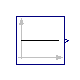Modelica.Blocks.Sources.Constant

Generate constant signal of type Real

### Information

The Real output y is a constant signal:Extends from Interfaces.SO (Single Output continuous control block).

### Parameters

NameDescription
kConstant output value

### Connectors

NameDescription
yConnector of Real output signal

##Modelica.Blocks.Sources.Step

Generate step signal of type Real

### Information

The Real output y is a step signal:Extends from Interfaces.SignalSource (Base class for continuous signal source).

### Parameters

NameDescription
heightHeight of step
offsetOffset of output signal y
startTimeOutput y = offset for time < startTime [s]

### Connectors

NameDescription
yConnector of Real output signal

##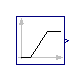Modelica.Blocks.Sources.Ramp

Generate ramp signal

### Information

The Real output y is a ramp signal:If parameter duration is set to 0.0, the limiting case of a Step signal is achieved.

Extends from Interfaces.SO (Single Output continuous control block).

### Parameters

NameDescription
heightHeight of ramps
durationDuration of ramp (= 0.0 gives a Step) [s]
offsetOffset of output signal
startTimeOutput = offset for time < startTime [s]

### Connectors

NameDescription
yConnector of Real output signal

##Modelica.Blocks.Sources.Sine

Generate sine signal

### Information

The Real output y is a sine signal:Extends from Interfaces.SO (Single Output continuous control block).

### Parameters

NameDescription
amplitudeAmplitude of sine wave
freqHzFrequency of sine wave [Hz]
offsetOffset of output signal
startTimeOutput = offset for time < startTime [s]

### Connectors

NameDescription
yConnector of Real output signal

##Modelica.Blocks.Sources.Cosine

Generate cosine signal

### Information

The Real output y is a cosine signal:Extends from Interfaces.SO (Single Output continuous control block).

### Parameters

NameDescription
amplitudeAmplitude of cosine wave
freqHzFrequency of cosine wave [Hz]
offsetOffset of output signal
startTimeOutput = offset for time < startTime [s]

### Connectors

NameDescription
yConnector of Real output signal

##Modelica.Blocks.Sources.ExpSine

Generate exponentially damped sine signal

### Information

The Real output y is a sine signal with exponentially changing amplitude:Extends from Interfaces.SO (Single Output continuous control block).

### Parameters

NameDescription
amplitudeAmplitude of sine wave
freqHzFrequency of sine wave [Hz]
dampingDamping coefficient of sine wave [s-1]
offsetOffset of output signal
startTimeOutput = offset for time < startTime [s]

### Connectors

NameDescription
yConnector of Real output signal

##Modelica.Blocks.Sources.Exponentials

Generate a rising and falling exponential signal

### Information

The Real output y is a rising exponential followed by a falling exponential signal:Extends from Interfaces.SO (Single Output continuous control block).

### Parameters

NameDescription
outMaxHeight of output for infinite riseTime
riseTimeRise time [s]
riseTimeConstRise time constant; rising is defined as outMax*(1-exp(-riseTime/riseTimeConst)) [s]
fallTimeConstFall time constant [s]
offsetOffset of output signal
startTimeOutput = offset for time < startTime [s]

### Connectors

NameDescription
yConnector of Real output signal

##Modelica.Blocks.Sources.Pulse

Generate pulse signal of type Real

### Information

The Real output y is a pulse signal:Extends from Modelica.Blocks.Interfaces.SO (Single Output continuous control block).

### Parameters

NameDescription
amplitudeAmplitude of pulse
widthWidth of pulse in % of period
periodTime for one period [s]
nperiodNumber of periods (< 0 means infinite number of periods)
offsetOffset of output signals
startTimeOutput = offset for time < startTime [s]

### Connectors

NameDescription
yConnector of Real output signal

##Modelica.Blocks.Sources.SawTooth

Generate saw tooth signal

### Information

The Real output y is a saw tooth signal:Extends from Interfaces.SO (Single Output continuous control block).

### Parameters

NameDescription
amplitudeAmplitude of saw tooth
periodTime for one period [s]
nperiodNumber of periods (< 0 means infinite number of periods)
offsetOffset of output signals
startTimeOutput = offset for time < startTime [s]

### Connectors

NameDescription
yConnector of Real output signal

##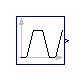Modelica.Blocks.Sources.Trapezoid

Generate trapezoidal signal of type Real

### Information

The Real output y is a trapezoid signal:Extends from Interfaces.SO (Single Output continuous control block).

### Parameters

NameDescription
amplitudeAmplitude of trapezoid
risingRising duration of trapezoid [s]
widthWidth duration of trapezoid [s]
fallingFalling duration of trapezoid [s]
periodTime for one period [s]
nperiodNumber of periods (< 0 means infinite number of periods)
offsetOffset of output signal
startTimeOutput = offset for time < startTime [s]

### Connectors

NameDescription
yConnector of Real output signal

##Modelica.Blocks.Sources.KinematicPTP

Move as fast as possible along a distance within given kinematic constraints

### Information

The goal is to move as fast as possible along a distance deltaq under given kinematical constraints. The distance can be a positional or angular range. In robotics such a movement is called PTP (Point-To-Point). This source block generates the acceleration qdd of this signal as output:After integrating the output two times, the position q is obtained. The signal is constructed in such a way that it is not possible to move faster, given the maximally allowed velocity qd_max and the maximally allowed acceleration qdd_max.

If several distances are given (vector deltaq has more than 1 element), an acceleration output vector is constructed such that all signals are in the same periods in the acceleration, constant velocity and deceleration phase. This means that only one of the signals is at its limits whereas the others are synchronized in such a way that the end point is reached at the same time instant.

This element is useful to generate a reference signal for a controller which controls a drive train or in combination with model Modelica.Mechanics.Rotational.Accelerate to drive a flange according to a given acceleration.

Extends from Interfaces.MO (Multiple Output continuous control block).

### Parameters

NameDescription
deltaq[:]Distance to move
qd_max[:]Maximum velocities der(q)
qdd_max[:]Maximum accelerations der(qd)
startTimeTime instant at which movement starts [s]
noutNumber of outputs

### Connectors

NameDescription
y[nout]Connector of Real output signals

##Modelica.Blocks.Sources.KinematicPTP2

Move as fast as possible from start to end position within given kinematic constraints with output signals q, qd=der(q), qdd=der(qd)

### Information

The goal is to move as fast as possible from start position q_begin to end position q_end under given kinematical constraints. The positions can be translational or rotational definitions (i.e., q_begin/q_end is given). In robotics such a movement is called PTP (Point-To-Point). This source block generates the position q(t), the speed qd(t) = der(q), and the acceleration qdd = der(qd) as output. The signals are constructed in such a way that it is not possible to move faster, given the maximally allowed velocity qd_max and the maximally allowed acceleration qdd_max:If vectors q_begin/q_end have more than 1 element, the output vectors are constructed such that all signals are in the same periods in the acceleration, constant velocity and deceleration phase. This means that only one of the signals is at its limits whereas the others are synchronized in such a way that the end point is reached at the same time instant.

This element is useful to generate a reference signal for a controller which controls, e.g., a drive train, or to drive a flange according to a given acceleration.

Extends from Modelica.Blocks.Icons.Block (Basic graphical layout of input/output block).

### Parameters

NameDescription
q_begin[:]Start position
q_end[:]End position
qd_max[:]Maximum velocities der(q)
qdd_max[:]Maximum accelerations der(qd)
startTimeTime instant at which movement starts [s]

### Connectors

NameDescription
q[nout]Reference position of path planning
qd[nout]Reference speed of path planning
qdd[nout]Reference acceleration of path planning
moving[nout]= true, if end position not yet reached; = false, if end position reached or axis is completely at rest

##Modelica.Blocks.Sources.TimeTable

Generate a (possibly discontinuous) signal by linear interpolation in a table

### Information

This block generates an output signal by linear interpolation in a table. The time points and function values are stored in a matrix `table[i,j]`, where the first column table[:,1] contains the time points and the second column contains the data to be interpolated. The table interpolation has the following properties:

• The time points need to be monotonically increasing.
• Discontinuities are allowed, by providing the same time point twice in the table.
• Values outside of the table range, are computed by extrapolation through the last or first two points of the table.
• If the table has only one row, no interpolation is performed and the function value is just returned independently of the actual time instant.
• Via parameters `startTime` and `offset` the curve defined by the table can be shifted both in time and in the ordinate value.
• The first point in time always has to be set to `0`, e.g., `table=[1,1;2,2]` is illegal. If you want to shift the time table in time use the `startTime` parameter instead.
• The table is implemented in a numerically sound way by generating time events at interval boundaries. This generates continuously differentiable values for the integrator.

Example:

```   table = [0  0
1  0
1  1
2  4
3  9
4 16]
If, e.g., time = 1.0, the output y =  0.0 (before event), 1.0 (after event)
e.g., time = 1.5, the output y =  2.5,
e.g., time = 2.0, the output y =  4.0,
e.g., time = 5.0, the output y = 23.0 (i.e., extrapolation).
```Extends from Interfaces.SO (Single Output continuous control block).

### Parameters

NameDescription
table[:, 2]Table matrix (time = first column; e.g., table=[0, 0; 1, 1; 2, 4])
offsetOffset of output signal
startTimeOutput = offset for time < startTime [s]

### Connectors

NameDescription
yConnector of Real output signal

##Modelica.Blocks.Sources.CombiTimeTable

Table look-up with respect to time and linear/periodic extrapolation methods (data from matrix/file)

### Information

This block generates an output signal y[:] by linear interpolation in a table. The time points and function values are stored in a matrix table[i,j], where the first column table[:,1] contains the time points and the other columns contain the data to be interpolated.Via parameter columns it can be defined which columns of the table are interpolated. If, e.g., columns={2,4}, it is assumed that 2 output signals are present and that the first output is computed by interpolation of column 2 and the second output is computed by interpolation of column 4 of the table matrix. The table interpolation has the following properties:

• The time points need to be strictly increasing if smoothness is ContinuousDerivative, otherwise monotonically increasing.
• Discontinuities are allowed, by providing the same time point twice in the table.
• Values outside of the table range, are computed by extrapolation according to the setting of parameter extrapolation:
```  extrapolation = 1: hold the first or last value of the table,
if outside of the table scope.
= 2: extrapolate by using the derivative at the first/last table
points if outside of the table scope.
(If smoothness is LinearSegments or ConstantSegments
this means to extrapolate linearly through the first/last
two table points.).
= 3: periodically repeat the table data
(periodical function).
= 4: no extrapolation, i.e. extrapolation triggers an error
```
• Via parameter smoothness it is defined how the data is interpolated:
```  smoothness = 1: linear interpolation
= 2: smooth interpolation with Akima-splines such
that der(y) is continuous, also if extrapolated.
= 3: constant segments
```
• If the table has only one row, no interpolation is performed and the table values of this row are just returned.
• Via parameters startTime and offset the curve defined by the table can be shifted both in time and in the ordinate value. The time instants stored in the table are therefore relative to startTime. If time < startTime, no interpolation is performed and the offset is used as ordinate value for all outputs.
• The table is implemented in a numerically sound way by generating time events at interval boundaries. This generates continuously differentiable values for the integrator.
• For special applications it is sometimes needed to know the minimum and maximum time instant defined in the table as a parameter. For this reason parameters t_min and t_max are provided and can be accessed from the outside of the table object.

Example:

```   table = [0  0
1  0
1  1
2  4
3  9
4 16]; extrapolation = 3 (default)
If, e.g., time = 1.0, the output y =  0.0 (before event), 1.0 (after event)
e.g., time = 1.5, the output y =  2.5,
e.g., time = 2.0, the output y =  4.0,
e.g., time = 5.0, the output y = 23.0 (i.e., extrapolation via last 2 points).
```

The table matrix can be defined in the following ways:

1. Explicitly supplied as parameter matrix "table", and the other parameters have the following values:
```   tableName is "NoName" or has only blanks,
fileName  is "NoName" or has only blanks.
```
2. Read from a file "fileName" where the matrix is stored as "tableName". Both ASCII and MAT-file format is possible. (The ASCII format is described below). The MAT-file format comes in four different versions: v4, v6, v7 and v7.3. The library supports at least v4, v6 and v7 whereas v7.3 is optional. It is most convenient to generate the MAT-file from FreeMat or MATLAB® by command
```   save tables.mat tab1 tab2 tab3
```
or Scilab by command
```   savematfile tables.mat tab1 tab2 tab3
```
when the three tables tab1, tab2, tab3 should be used from the model.
Note, a fileName can be defined as URI by using the helper function loadResource.
3. Statically stored in function "usertab" in file "usertab.c". The matrix is identified by "tableName". Parameter fileName = "NoName" or has only blanks. Row-wise storage is always to be preferred as otherwise the table is reallocated and transposed.

When the constant "NO_FILE_SYSTEM" is defined, all file I/O related parts of the source code are removed by the C-preprocessor, such that no access to files takes place.

If tables are read from an ASCII-file, the file needs to have the following structure ("-----" is not part of the file content):

```-----------------------------------------------------
#1
double tab1(6,2)   # comment line
0   0
1   0
1   1
2   4
3   9
4  16
double tab2(6,2)   # another comment line
0   0
2   0
2   2
4   8
6  18
8  32
-----------------------------------------------------
```

Note, that the first two characters in the file need to be "#1" (a line comment defining the version number of the file format). Afterwards, the corresponding matrix has to be declared with type (= "double" or "float"), name and actual dimensions. Finally, in successive rows of the file, the elements of the matrix have to be given. The elements have to be provided as a sequence of numbers in row-wise order (therefore a matrix row can span several lines in the file and need not start at the beginning of a line). Numbers have to be given according to C syntax (such as 2.3, -2, +2.e4). Number separators are spaces, tab ( ), comma (,), or semicolon (;). Several matrices may be defined one after another. Line comments start with the hash symbol (#) and can appear everywhere. Other characters, like trailing non comments, are not allowed in the file.

MATLAB is a registered trademark of The MathWorks, Inc.

Extends from Modelica.Blocks.Interfaces.MO (Multiple Output continuous control block).

### Parameters

NameDescription
noutNumber of outputs
Table data definition
tableOnFile= true, if table is defined on file or in function usertab
table[:, :]Table matrix (time = first column; e.g., table=[0,2])
tableNameTable name on file or in function usertab (see docu)
fileNameFile where matrix is stored
Table data interpretation
columns[:]Columns of table to be interpolated
smoothnessSmoothness of table interpolation
extrapolationExtrapolation of data outside the definition range
offset[:]Offsets of output signals
startTimeOutput = offset for time < startTime [s]

### Connectors

NameDescription
y[nout]Connector of Real output signals

##Modelica.Blocks.Sources.BooleanConstant

Generate constant signal of type Boolean

### Information

The Boolean output y is a constant signal:Extends from Interfaces.partialBooleanSource (Partial source block (has 1 output Boolean signal and an appropriate default icon)).

### Parameters

NameDescription
kConstant output value

### Connectors

NameDescription
yConnector of Boolean output signal

##Modelica.Blocks.Sources.BooleanStep

Generate step signal of type Boolean

### Information

The Boolean output y is a step signal:Extends from Interfaces.partialBooleanSource (Partial source block (has 1 output Boolean signal and an appropriate default icon)).

### Parameters

NameDescription
startTimeTime instant of step start [s]
startValueOutput before startTime

### Connectors

NameDescription
yConnector of Boolean output signal

##Modelica.Blocks.Sources.BooleanPulse

Generate pulse signal of type Boolean

### Information

The Boolean output y is a pulse signal:Extends from Modelica.Blocks.Interfaces.partialBooleanSource (Partial source block (has 1 output Boolean signal and an appropriate default icon)).

### Parameters

NameDescription
widthWidth of pulse in % of period
periodTime for one period [s]
startTimeTime instant of first pulse [s]

### Connectors

NameDescription
yConnector of Boolean output signal

##Modelica.Blocks.Sources.SampleTrigger

Generate sample trigger signal

### Information

The Boolean output y is a trigger signal where the output y is only true at sample times (defined by parameter period) and is otherwise false.Extends from Interfaces.partialBooleanSource (Partial source block (has 1 output Boolean signal and an appropriate default icon)).

### Parameters

NameDescription
periodSample period [s]
startTimeTime instant of first sample trigger [s]

### Connectors

NameDescription
yConnector of Boolean output signal

##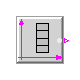Modelica.Blocks.Sources.BooleanTable

Generate a Boolean output signal based on a vector of time instants

### Information

The Boolean output y is a signal defined by parameter vector table. In the vector time points are stored. At every time point, the output y changes its value to the negated value of the previous one.Extends from Interfaces.partialBooleanSource (Partial source block (has 1 output Boolean signal and an appropriate default icon)).

### Parameters

NameDescription
startValueStart value of y. At time = table, y changes to 'not startValue'
table[:]Vector of time points. At every time point, the output y gets its opposite value (e.g., table={0,1}) [s]

### Connectors

NameDescription
yConnector of Boolean output signal

##Modelica.Blocks.Sources.RadioButtonSource

Boolean signal source that mimics a radio button

### Information

Boolean signal source that mimics a radio button: Via a table, a radio button is pressed (i.e., the output 'on' is set to true) and is reset when an element of the Boolean vector 'reset' becomes true. If both appear at the same time instant, setting the button according to the table has a higher priority as resetting the button. Example:

```  RadioButtonSource start(buttonTimeTable={1,3}, reset={stop.on});
```

The "start" button is pressed at time=1 s and time=3 s, whereas the "stop" button is pressed at time=2 s and time=4 s. This gives the following result:This example is also available in Modelica.Blocks.Examples.Interaction1

### Parameters

NameDescription
buttonTimeTable[:]Time instants where button is pressed [s]
Time varying expressions
reset[:]Reset button to false, if an element of reset becomes true

NameDescription
on

##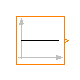Modelica.Blocks.Sources.IntegerConstant

Generate constant signal of type Integer

### Information

The Integer output y is a constant signal:Extends from Interfaces.IntegerSO (Single Integer Output continuous control block).

### Parameters

NameDescription
kConstant output value

### Connectors

NameDescription
yConnector of Integer output signal

##Modelica.Blocks.Sources.IntegerStep

Generate step signal of type Integer

### Information

The Integer output y is a step signal:Extends from Interfaces.IntegerSignalSource (Base class for continuous Integer signal source).

### Parameters

NameDescription
heightHeight of step
offsetOffset of output signal y
startTimeOutput y = offset for time < startTime [s]

### Connectors

NameDescription
yConnector of Integer output signal

##Modelica.Blocks.Sources.IntegerTable

Generate an Integer output signal based on a table matrix with [time, yi] values

### Information

This block generates an Integer output signal by using a table. The time points and y-values are stored in a matrix table[i,j], where the first column table[:,1] contains the Real time points and the second column contains the Integer value of the output y at this time point.

An assert is triggered, if no table values are provided, if the time points are not strict monotonically increasing, or if the second column of the table matrix does not contain Integer values.

If the simulation time is less than the first table time instant, then the output y = table[1,2].
If the simulation time is greater than the last table time instant, then the output y = table[end,2].

Example:

```   table = [  0, 1;
1, 4;
1.5, 5;
2, 6];
```

results in the following output:Extends from Interfaces.IntegerSO (Single Integer Output continuous control block).

### Parameters

NameDescription
table[:, 2]Table matrix (first column: time; second column: y)

### Connectors

NameDescription
yConnector of Integer output signal

Automatically generated Mon Sep 23 17:20:25 2013.